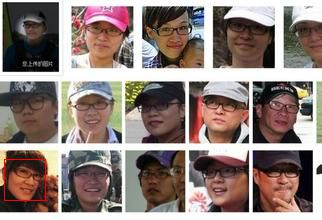# python+opencv实现的简单人脸识别代码示例

更新时间：2017年11月14日 09:32:38   作者：决心1119我要评论

#

```#!/usr/bin/env python
#coding=utf-8
import os
from PIL import Image, ImageDraw
import cv
def detect_object(image):
'''检测图片，获取人脸在图片中的坐标'''
grayscale = cv.CreateImage((image.width, image.height), 8, 1)
cv.CvtColor(image, grayscale, cv.CV_BGR2GRAY)
rect = cv.HaarDetectObjects(grayscale, cascade, cv.CreateMemStorage(), 1.1, 2,
cv.CV_HAAR_DO_CANNY_PRUNING, (20,20))
result = []
for r in rect:
result.append((r, r, r+r, r+r))
return result
def process(infile):
'''在原图上框出头像并且截取每个头像到单独文件夹'''
if image:
faces = detect_object(image)
im = Image.open(infile)
path = os.path.abspath(infile)
save_path = os.path.splitext(path)+"_face"
try:
os.mkdir(save_path)
except:
pass
if faces:
draw = ImageDraw.Draw(im)
count = 0
for f in faces:
count += 1
draw.rectangle(f, outline=(255, 0, 0))
drow_save_path = os.path.join(save_path,"out.jpg")
im.save(drow_save_path, "JPEG", quality=80)
else:
print "Error: cannot detect faces on %s" % infile
if __name__ == "__main__":
process("/Users/zhangdebin/Documents/checkFace2.jpg")```python图像常规操作

Python生成数字图片代码分享